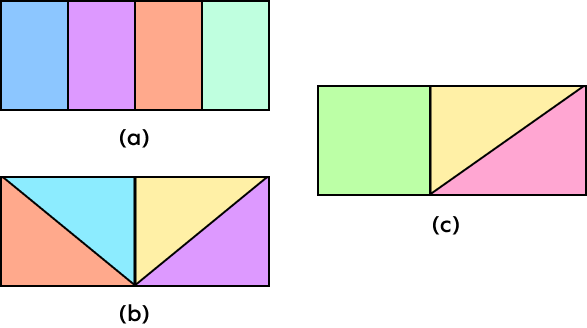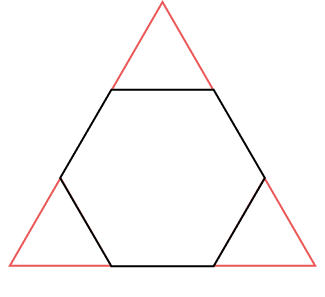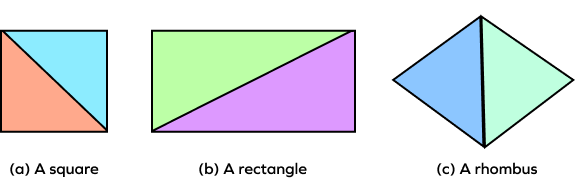# Composing Shapes – Definition with Examples

## Introduction

The word compose means to combine, create, or put together. In our daily life, we come across several geometric shapes. Have you ever combined two or more geometric shapes to form a new shape? For instance, have you taken two squares and joined them to form a rectangle? It must have seemed like a fun activity, wasn’t it? Well, such activities involving shapes can teach us several important concepts in geometry.

Today, we shall learn more about the concept of composing shapes. So, let’s get started right away!

## What Does Composing 2D Shapes mean?

Composing shapes means combining two or more simple shapes to create a larger shape. Simple shapes in geometry include triangles, circles, squares, rectangles, etc. While composing shapes, you can combine any of these shapes. You can use the same or different shapes to construct a new shape. You can even use sections of different shapes for this purpose.

## Examples of Composing Shapes

Figure 1 shows two triangles combined to form a square. Here, two shapes that are the same have been used to create a new shape.

Fig: 1

Figure 2 shows a shape composed by combining a square and a triangle. To make this new shape, two different shapes have been used.

Fig: 2

Figure 3 shows a hexagonal shape formed by using three simple shapes. Among the simple shapes used here, two are triangles, and one is a square.

Fig: 3

Figure 4 shows a heart formed by a square and two semicircles. Here, sections of a circle are combined with a square to form the new shape.

Fig: 4

Fun Fact!

A rectangle, a square, and a triangle may seem like three simple shapes. But they can combine to become a cute house, as shown in figure 5 below.

Fig: 5

## Conclusion

Knowing how to compose shapes is an essential step for learning advanced math concepts, such as area, proportions, and fractions. Composing shapes helps in identifying and organizing visual information. It sets the stage for advanced geometric concepts.

## Solved Examples

### Example 1: Name two simple shapes that you can use to compose the figure below?

Solution: The two simple shapes that we can use to compose the given figure are a triangle and a semicircle.

If we take a semicircle and a triangle, and place the semicircle in a way that it touches one of the sides of the triangle, we can get the shape shown here.

### Example 2: How can you compose an arrow shape using the shapes given below?

Solution: The given shapes are a triangle and a rectangle. We can compose an arrow shape using the given shapes as shown below:

### Example 3:  Is it possible to compose a hexagon with the given shapes?

Solution: Yes, it is possible to compose a hexagon with the given shapes.

If we arrange the shapes as shown in the figure below, we can create a hexagon using them.

### Example 4: Can we form a pentagon using the two shapes given below?

Solution: Yes, we can form a pentagon using the given shapes. We can arrange the given shapes as shown below to form a pentagon:

## Practice Problems

1

### Which of the following shapes can you use to create a rectangle?

Four rectangles
Four triangles
A square and two triangles
All of the above
CorrectIncorrect
Correct answer is: All of the above
The figures below show how we can use the given shapes to create a rectangle.2

### What shape can you form using the given figures?A triangle
A circle
An oval
None of the above
CorrectIncorrect
Since the given figures have no curved edges, we can’t create a circle or an oval using them. But if we arrange the given figures as shown below, we can create a triangle.3

### Which of the following shapes can be formed using two equal triangles?

A square
A rectangle
A rhombus
All of the above
CorrectIncorrect
Correct answer is: All of the above
If we arrange a pair of equal triangles as shown in the figures below, we can create a square, a rectangle, and a rhombus using them.